Question

# How much soda ash (Na2CO3) in kg/day do you need to add to remove calcium hardness...

How much soda ash (Na2CO3) in kg/day do you need to add to remove calcium hardness from 200 mg/L CaCO3 to 50 mg/L CaCO3 if you have a water source with flowrate of 1000 m3/day. Consider the following unbalanced reaction that describes the process of Ca2+ removal. Ca2+ + Na2CO3 ↔ CaCO3↓ + Na+ Considering the soda ash has 96% purity and it costs \$0.5/kg, how much would it cost the water treatment plant per year (365 days)?

Here is the Solution:Thanks! :-)

#### Earn Coins

Coins can be redeemed for fabulous gifts.

Similar Homework Help Questions
• ### 3. Determine the lime and soda ash dose, in mg/L as Ca(OH)2 and Na2CO3, to soften...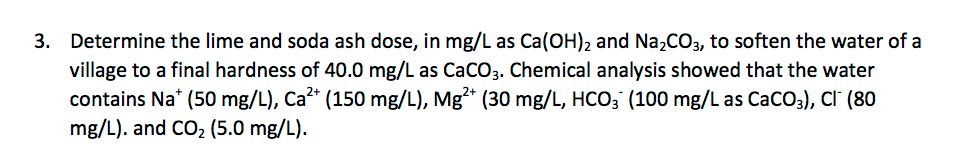3. Determine the lime and soda ash dose, in mg/L as Ca(OH)2 and Na2CO3, to soften the water of a village to a final hardness of 40.0 mg/L as CaCO3. Chemical analysis showed that the water contains Na+ (50 mg/L), Ca2+ (150 mg/L), Mg²+ (30 mg/L, HCO3 (100 mg/Las CaCO3), Cl (80 mg/L). and CO2 (5.0 mg/L).

• ### The chemical composition of a water sample is given in the table below. The goal hardness...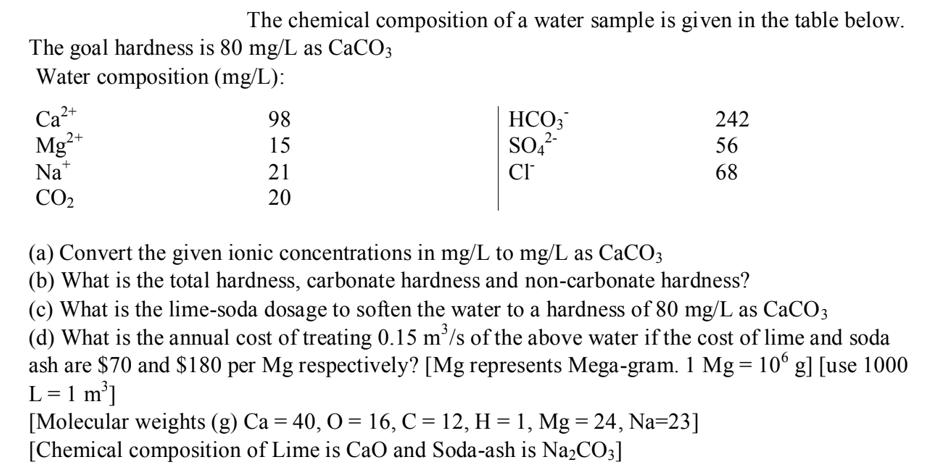The chemical composition of a water sample is given in the table below. The goal hardness is 80 mg/L as CaCO3 Water composition (mg/L): Ca2+ HCO3 15 SO42- Na* CO2 242 Mg2+ 56 (a) Convert the given ionic concentrations in mg/L to mg/L as CaCO3 (b) What is the total hardness, carbonate hardness and non-carbonate hardness? (C) What is the lime-soda dosage to soften the water to a hardness of 80 mg/L as CaCO3 (d) What is the annual cost...

• ### . 5 points. Using Eq. 4-17 (shown below) calculate the dose of calcium hydroxide in mg...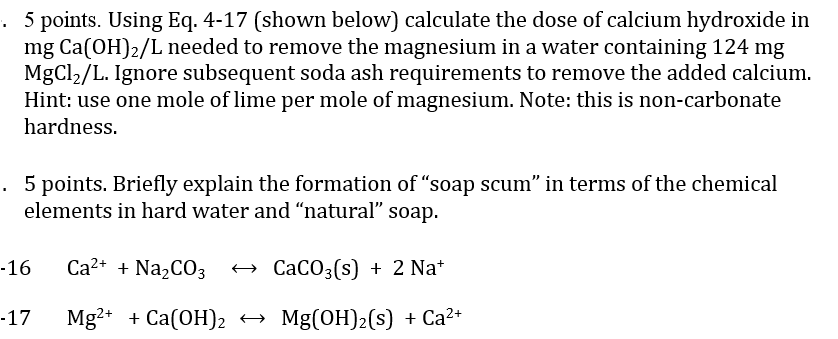. 5 points. Using Eq. 4-17 (shown below) calculate the dose of calcium hydroxide in mg Ca(OH)2/L needed to remove the magnesium in a water containing 124 mg MgCl2/L. Ignore subsequent soda ash requirements to remove the added calcium Hint: use one mole of lime per mole of magnesium. Note: this is non-carbonate hardness . 5 points. Briefly explain the formation of "soap scum" in terms of the chemical elements in hard water and "natural" soap. Ca2* Na2CO3 CaCO3(s)2 Na*...

• ### The following water quality data are available for a community Water quality Ca2+ = 60 mg/l...

The following water quality data are available for a community Water quality Ca2+ = 60 mg/l (as species) Mg2+ = 40 mg/l (as species) HCO3- = 220 mg/l as CaCO3 CO2 = 7 mg/l (as species) pH = 7.6 Final desired hardness = 80 mg/l as CaCO3. The flow rate of the treatment plant is 0.24 m3/s. a. Calculate the total, carbonate, and non-carbonate hardness b. Calculate the amount of lime (as mg/l of CaO) needed to soften the water...

• ### How much calcium bicarbonate (kg/day) will need to be added per day in water with alkalinity...

How much calcium bicarbonate (kg/day) will need to be added per day in water with alkalinity of 30 mg/L CaCO3 (assume for simplicity only bicarbonate alkalinity is present) if 794 kg of Al2(SO4)3 x18H2O is added per week to treat water with coagulation/flocculation in a water treatment plant with capacity of 1 MGD. Consider the following coagulation/flocculation (unbalanced) reactions occur: Al3+ + HCO3– → Al(OH)3 + CO2

• ### Please need ASAP Problem 1 (25 pts) The influent water to a water treatment plant that treats 50x10° L/day has a pH of 7.5 and the following composition: eqmolW (g/mol) [1 | (me/L) | [1 (mg/L...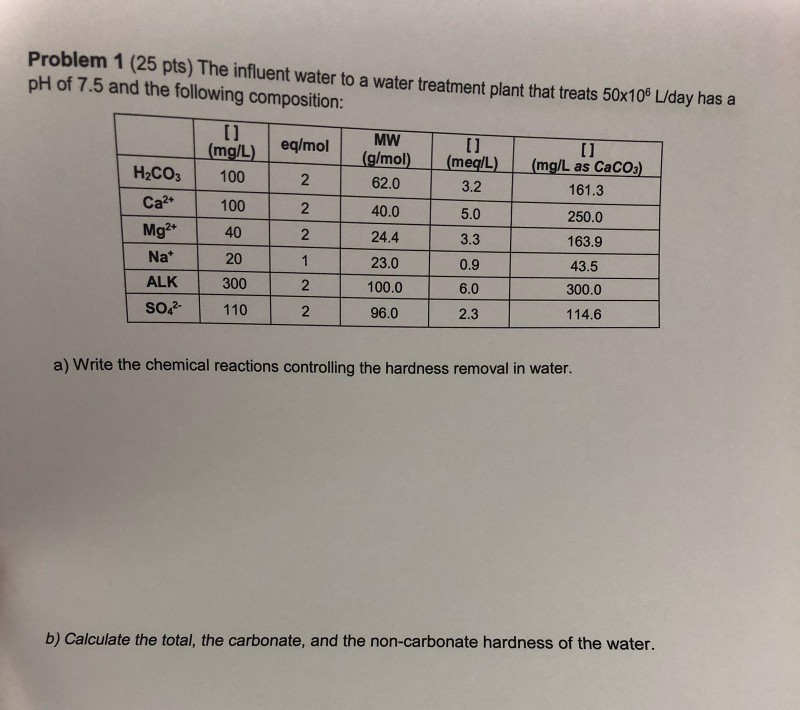Please need ASAP Problem 1 (25 pts) The influent water to a water treatment plant that treats 50x10° L/day has a pH of 7.5 and the following composition: eqmolW (g/mol) [1 | (me/L) | [1 (mg/L as CaCO) L) mg/L) | H2COs 100 2 62.0 3.2 161.3 Ca2 100 2 40.0 5.0 250.0 Mg2 40 2 24.4 3.3 163.9 Nat 43.5 20 0.9 23.0 300.0 300 100.0 6.0 ALK 114.6 2.3 96.0 110 SO1 a) Write the chemical reactions controlling...

• ### 2. Calculate the amount of lime as Ca(OH)2 (90% pure) and soda ash (93% pure) (each...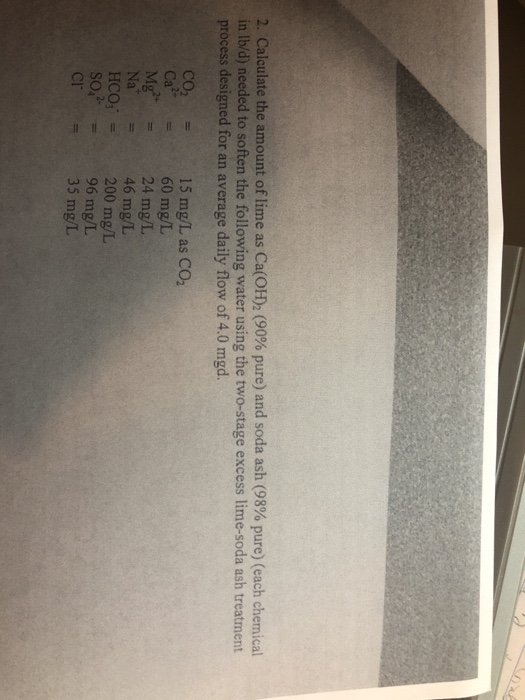2. Calculate the amount of lime as Ca(OH)2 (90% pure) and soda ash (93% pure) (each chemical in lb/d) needed to soften the following water using the two-stage excess lime-soda ash treatment process designed for an average daily flow of 4.0 mgd. 15 mg/L as CO2 CO2 Ca2+ 60 mg/L 24 mg/L Na 46 mg/L HCO3 = 200 mg/L 96 mg/L 35 mg/L = CF

• ### Question 30 (4 points) Groundwater defined by the following analysis is to be softened by lime-soda...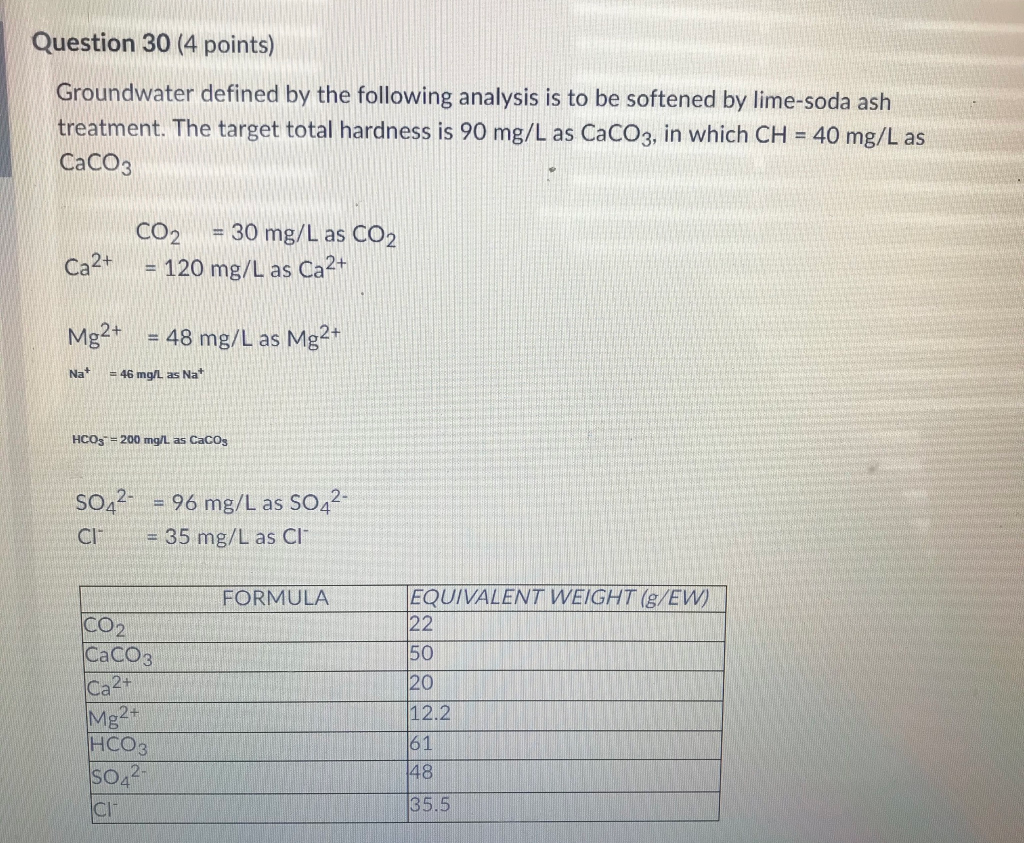Question 30 (4 points) Groundwater defined by the following analysis is to be softened by lime-soda ash treatment. The target total hardness is 90 mg/L as CaCO3, in which CH = 40 mg/L as CaCO3 CO2 = 30 mg/L as CO2 = 120 mg/L as Ca2+ Ca2+ Mg2+ = 48 mg/L as Mg2+ Nat = 46 mg/L as Nat HCO3 = 200 mg/L as Cacos SO42- 96 mg/L as SO42- 35 mg/L as CI FORMULA CO2 CaCO3 EQUIVALENT WEIGHT (S/EW)...

• ### 3. 10 points. Calculate the amounts (kg, 100% basis) of H2SO4 and NaOH required in a dual bed ion exchange system to re...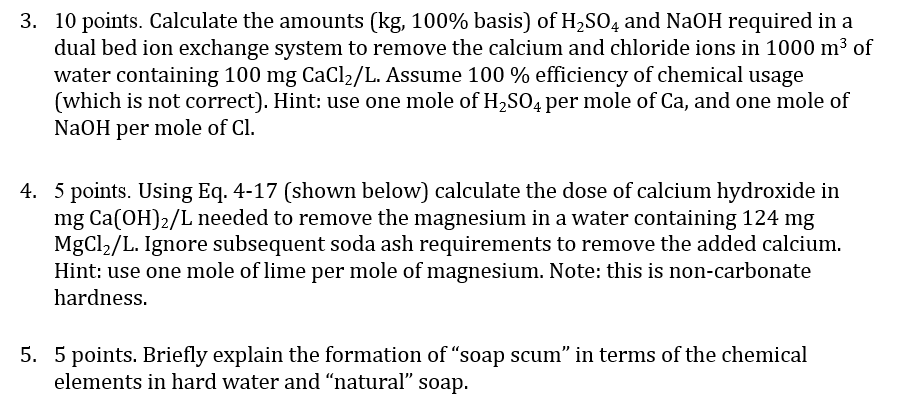3. 10 points. Calculate the amounts (kg, 100% basis) of H2SO4 and NaOH required in a dual bed ion exchange system to remove the calcium and chloride ions in 1000 m3 of water containing 100 mg CaCl2/L. Assume 100 % efficiency of chemical usage (which is not correct). Hint: use one mole of H2SO4 per mole of Ca, and one mole of NaOH per mole of Cl 4. 5 points. Using Eq. 4-17 (shown below) calculate the dose of calcium...

• ### Drinking water treatment plants often add powdered activated carbon (PAC) to remove natural organic matter (NOM)...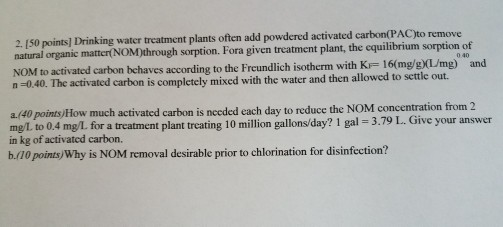Drinking water treatment plants often add powdered activated carbon (PAC) to remove natural organic matter (NOM) through sorption. For a given treatment plant, the equilibrium sorption of NOM to activated carbon behaves according to the Freundlich isotherm with K_F = 16 (mg/g) (L/mg)^0.40 and n = 0.40. The activated carbon is completely mixed with the water and then allowed to settle out. a. How much activated carbon is needed each day to reduce the NOM concentration from 2 mg/L to...